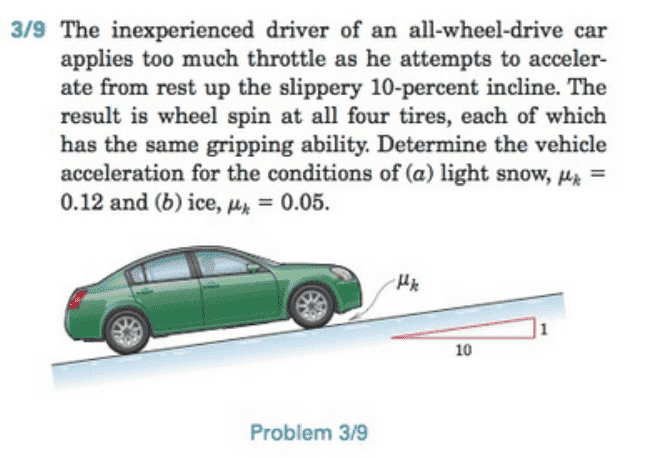# Kinetic Friction and Incline for a Car

## Homework Statement## Homework Equations

friction:[/B] f = μN
incline angle: θ = arctan(1/10)
summation of forces in y: N - mgcosθ = 0
summation of forces in x: -f - mgsinθ = ma (?????)

## The Attempt at a Solution

For this problem, I set the x-axis going up the incline and the y-axis normal to said x-axis. When I drew the FBD, the part that did not make sense to me was the summation of forces in the x-axis. The question wants the car to accelerate UP the incline, but the two forces I draw for the FBD both point down the incline. One of the forces is the x-component of the weight, mgsinθ, and the other is the friction. I know the weight's x-component direction is right, so the wildcard is the friction. Isn't friction opposite to the direction of motion?